This book is archived and will be removed July 6, 2022. Please use the updated version.

Relativity

# 36 Length Contraction

### Learning Objectives

By the end of this section, you will be able to:

• Explain how simultaneity and length contraction are related.
• Describe the relation between length contraction and time dilation and use it to derive the length-contraction equation.

The length of the train car in (Figure) is the same for all the passengers. All of them would agree on the simultaneous location of the two ends of the car and obtain the same result for the distance between them. But simultaneous events in one inertial frame need not be simultaneous in another. If the train could travel at relativistic speeds, an observer on the ground would see the simultaneous locations of the two endpoints of the car at a different distance apart than observers inside the car. Measured distances need not be the same for different observers when relativistic speeds are involved.

People might describe distances differently, but at relativistic speeds, the distances really are different. (credit: “russavia”/Flickr)### Proper Length

Two observers passing each other always see the same value of their relative speed. Even though time dilation implies that the train passenger and the observer standing alongside the tracks measure different times for the train to pass, they still agree that relative speed, which is distance divided by elapsed time, is the same. If an observer on the ground and one on the train measure a different time for the length of the train to pass the ground observer, agreeing on their relative speed means they must also see different distances traveled.

The muon discussed in (Figure) illustrates this concept ((Figure)). To an observer on Earth, the muon travels at 0.950c for 7.05 μs from the time it is produced until it decays. Therefore, it travels a distance relative to Earth of: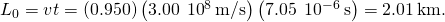In the muon frame, the lifetime of the muon is 2.20 μs. In this frame of reference, the Earth, air, and ground have only enough time to travel: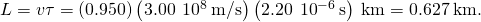The distance between the same two events (production and decay of a muon) depends on who measures it and how they are moving relative to it.

Proper Length

Proper length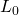is the distance between two points measured by an observer who is at rest relative to both of the points.

The earthbound observer measures the proper lengthbecause the points at which the muon is produced and decays are stationary relative to Earth. To the muon, Earth, air, and clouds are moving, so the distance L it sees is not the proper length.

(a) The earthbound observer sees the muon travel 2.01 km. (b) The same path has length 0.627 km seen from the muon’s frame of reference. The Earth, air, and clouds are moving relative to the muon in its frame, and have smaller lengths along the direction of travel.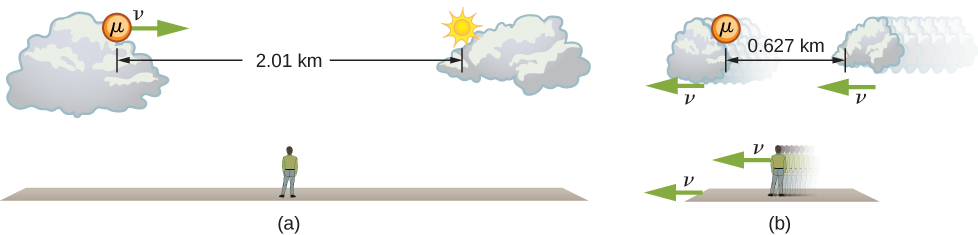### Length Contraction

To relate distances measured by different observers, note that the velocity relative to the earthbound observer in our muon example is given byThe time relative to the earthbound observer is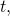because the object being timed is moving relative to this observer. The velocity relative to the moving observer is given by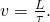The moving observer travels with the muon and therefore observes the proper time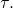The two velocities are identical; thus,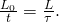We know that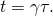Substituting this equation into the relationship above gives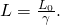Substituting for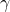gives an equation relating the distances measured by different observers.

Length Contraction

Length contraction is the decrease in the measured length of an object from its proper length when measured in a reference frame that is moving with respect to the object: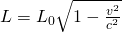whereis the length of the object in its rest frame, and L is the length in the frame moving with velocity v.

If we measure the length of anything moving relative to our frame, we find its length L to be smaller than the proper lengththat would be measured if the object were stationary. For example, in the muon’s rest frame, the distance Earth moves between where the muon was produced and where it decayed is shorter than the distance traveled as seen from the Earth’s frame. Those points are fixed relative to Earth but are moving relative to the muon. Clouds and other objects are also contracted along the direction of motion as seen from muon’s rest frame.

Thus, two observers measure different distances along their direction of relative motion, depending on which one is measuring distances between objects at rest.

But what about distances measured in a direction perpendicular to the relative motion? Imagine two observers moving along their x-axes and passing each other while holding meter sticks vertically in the y-direction. (Figure) shows two meter sticks M and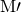that are at rest in the reference frames of two boys S and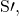respectively. A small paintbrush is attached to the top (the 100-cm mark) of stick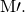Suppose that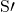is moving to the right at a very high speed v relative to S, and the sticks are oriented so that they are perpendicular, or transverse, to their relative velocity vector. The sticks are held so that as they pass each other, their lower ends (the 0-cm marks) coincide. Assume that when S looks at his stick M afterwards, he finds a line painted on it, just below the top of the stick. Because the brush is attached to the top of the other boy’s stick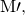S can only conclude that stickis less than 1.0 m long.

Meter sticks M andare stationary in the reference frames of observers S andrespectively. As the sticks pass, a small brush attached to the 100-cm mark ofpaints a line on M.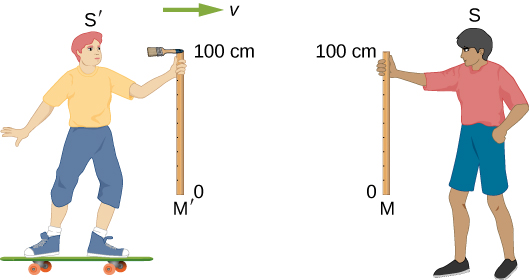Now when the boys approach each other,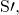like S, sees a meter stick moving toward him with speed v. Because their situations are symmetric, each boy must make the same measurement of the stick in the other frame. So, if S measures stickto be less than 1.0 m long,must measure stick M to be also less than 1.0 m long, andmust see his paintbrush pass over the top of stick M and not paint a line on it. In other words, after the same event, one boy sees a painted line on a stick, while the other does not see such a line on that same stick!

Einstein’s first postulate requires that the laws of physics (as, for example, applied to painting) predict that S andwho are both in inertial frames, make the same observations; that is, S andmust either both see a line painted on stick M, or both not see that line. We are therefore forced to conclude our original assumption that S saw a line painted below the top of his stick was wrong! Instead, S finds the line painted right at the 100-cm mark on M. Then both boys will agree that a line is painted on M, and they will also agree that both sticks are exactly 1 m long. We conclude then that measurements of a transverse length must be the same in different inertial frames.

Calculating Length Contraction Suppose an astronaut, such as the twin in the twin paradox discussion, travels so fast that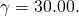(a) The astronaut travels from Earth to the nearest star system, Alpha Centauri, 4.300 light years (ly) away as measured by an earthbound observer. How far apart are Earth and Alpha Centauri as measured by the astronaut? (b) In terms of c, what is the astronaut’s velocity relative to Earth? You may neglect the motion of Earth relative to the sun ((Figure)).

(a) The earthbound observer measures the proper distance between Earth and Alpha Centauri. (b) The astronaut observes a length contraction because Earth and Alpha Centauri move relative to her ship. She can travel this shorter distance in a smaller time (her proper time) without exceeding the speed of light.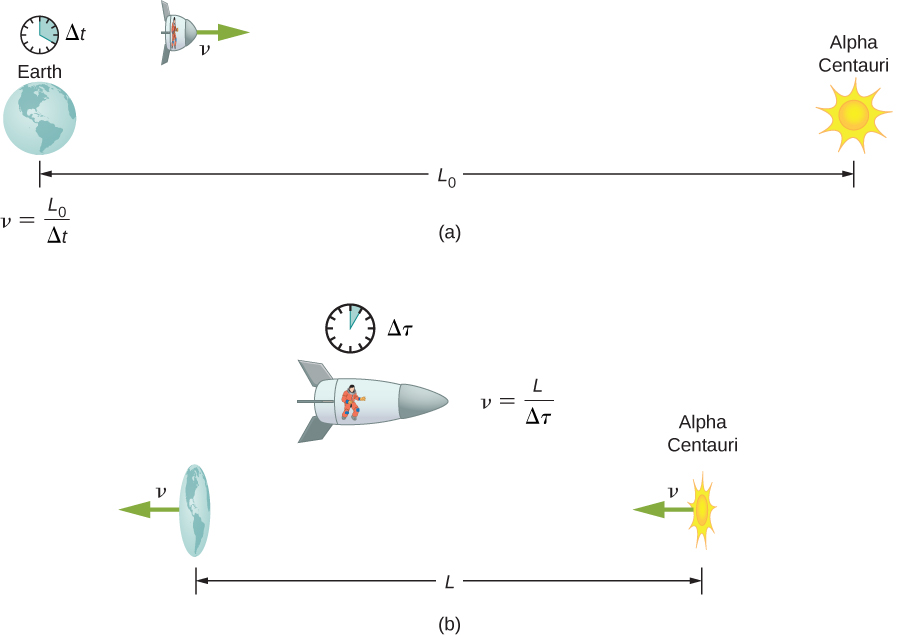Strategy First, note that a light year (ly) is a convenient unit of distance on an astronomical scale—it is the distance light travels in a year. For part (a), the 4.300-ly distance between Alpha Centauri and Earth is the proper distance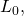because it is measured by an earthbound observer to whom both stars are (approximately) stationary. To the astronaut, Earth and Alpha Centauri are moving past at the same velocity, so the distance between them is the contracted length L. In part (b), we are given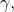so we can find v by rearranging the definition ofto express v in terms of c.

Solution for (a) For part (a):

1. Identify the knowns: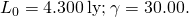2. Identify the unknown: L.
3. Express the answer as an equation:4. Do the calculation: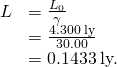Solution for (b) For part (b):

1. Identify the known:2. Identify the unknown: v in terms of c.Then solve for the unknown v/c by first squaring both sides and then rearranging: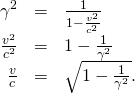4. Do the calculation: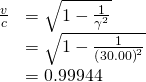or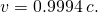Significance Remember not to round off calculations until the final answer, or you could get erroneous results. This is especially true for special relativity calculations, where the differences might only be revealed after several decimal places. The relativistic effect is large here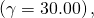and we see that v is approaching (not equaling) the speed of light. Because the distance as measured by the astronaut is so much smaller, the astronaut can travel it in much less time in her frame.

People traveling at extremely high velocities could cover very large distances (thousands or even millions of light years) and age only a few years on the way. However, like emigrants in past centuries who left their home, these people would leave the Earth they know forever. Even if they returned, thousands to millions of years would have passed on Earth, obliterating most of what now exists. There is also a more serious practical obstacle to traveling at such velocities; immensely greater energies would be needed to achieve such high velocities than classical physics predicts can be attained. This will be discussed later in the chapter.

Why don’t we notice length contraction in everyday life? The distance to the grocery store does not seem to depend on whether we are moving or not. Examining the equation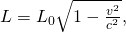we see that at low velocities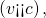the lengths are nearly equal, which is the classical expectation. But length contraction is real, if not commonly experienced. For example, a charged particle such as an electron traveling at relativistic velocity has electric field lines that are compressed along the direction of motion as seen by a stationary observer ((Figure)). As the electron passes a detector, such as a coil of wire, its field interacts much more briefly, an effect observed at particle accelerators such as the 3-km-long Stanford Linear Accelerator (SLAC). In fact, to an electron traveling down the beam pipe at SLAC, the accelerator and Earth are all moving by and are length contracted. The relativistic effect is so great that the accelerator is only 0.5 m long to the electron. It is actually easier to get the electron beam down the pipe, because the beam does not have to be as precisely aimed to get down a short pipe as it would to get down a pipe 3 km long. This, again, is an experimental verification of the special theory of relativity.

The electric field lines of a high-velocity charged particle are compressed along the direction of motion by length contraction, producing an observably different signal as the particle goes through a coil.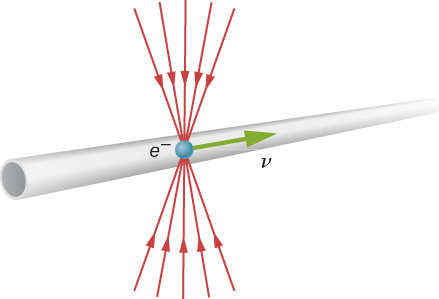Check Your Understanding A particle is traveling through Earth’s atmosphere at a speed of 0.750c. To an earthbound observer, the distance it travels is 2.50 km. How far does the particle travel as viewed from the particle’s reference frame?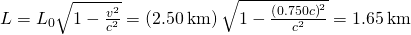### Summary

• All observers agree upon relative speed.
• Distance depends on an observer’s motion. Proper lengthis the distance between two points measured by an observer who is at rest relative to both of the points.
• Length contraction is the decrease in observed length of an object from its proper lengthto length L when its length is observed in a reference frame where it is traveling at speed v.
• The proper length is the longest measurement of any length interval. Any observer who is moving relative to the system being observed measures a length shorter than the proper length.

### Conceptual Questions

To whom does an object seem greater in length, an observer moving with the object or an observer moving relative to the object? Which observer measures the object’s proper length?

The length of an object is greatest to an observer who is moving with the object, and therefore measures its proper length.

Relativistic effects such as time dilation and length contraction are present for cars and airplanes. Why do these effects seem strange to us?

Suppose an astronaut is moving relative to Earth at a significant fraction of the speed of light. (a) Does he observe the rate of his clocks to have slowed? (b) What change in the rate of earthbound clocks does he see? (c) Does his ship seem to him to shorten? (d) What about the distance between two stars that lie in the direction of his motion? (e) Do he and an earthbound observer agree on his velocity relative to Earth?

a. No, not within the astronaut’s own frame of reference. b. He sees Earth clocks to be in their rest frame moving by him, and therefore sees them slowed. c. No, not within the astronaut’s own frame of reference. d. Yes, he measures the distance between the two stars to be shorter. e. The two observers agree on their relative speed.

### Problems

A spaceship, 200 m long as seen on board, moves by the Earth at 0.970c. What is its length as measured by an earthbound observer?

48.6 m

How fast would a 6.0 m-long sports car have to be going past you in order for it to appear only 5.5 m long?

(a) How far does the muon in (Figure) travel according to the earthbound observer? (b) How far does it travel as viewed by an observer moving with it? Base your calculation on its velocity relative to the Earth and the time it lives (proper time). (c) Verify that these two distances are related through length contraction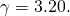Using the values given in (Figure): a. 1.39 km; b. 0.433 km; c. 0.433 km

(a) How long would the muon in (Figure) have lived as observed on Earth if its velocity was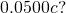(b) How far would it have traveled as observed on Earth? (c) What distance is this in the muon’s frame?

Unreasonable Results A spaceship is heading directly toward Earth at a velocity of 0.800c. The astronaut on board claims that he can send a canister toward the Earth at 1.20c relative to Earth. (a) Calculate the velocity the canister must have relative to the spaceship. (b) What is unreasonable about this result? (c) Which assumptions are unreasonable or inconsistent?

a. 10.0c; b. The resulting speed of the canister is greater than c, an impossibility. c. It is unreasonable to assume that the canister will move toward the earth at 1.20c.

### Glossary

length contraction
decrease in observed length of an object from its proper lengthto length L when its length is observed in a reference frame where it is traveling at speed v
proper length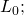the distance between two points measured by an observer who is at rest relative to both of the points; for example, earthbound observers measure proper length when measuring the distance between two points that are stationary relative to Earth